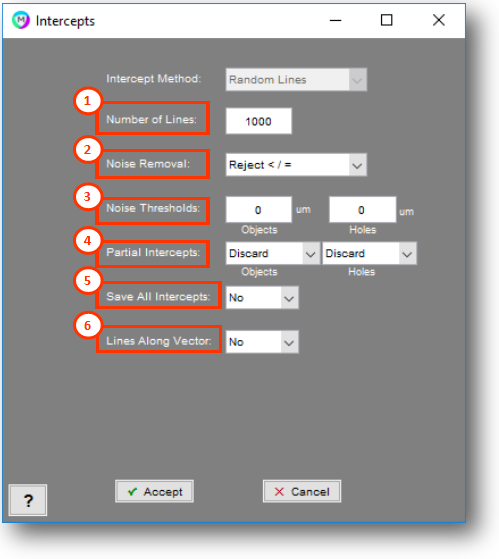## Random Lines

Measures metrics such as mean intercept by drawing a specified number of random lines through the features in the reconstruction.### 1. Number of Lines

Number of lines to draw

### 2. Noise Removal

Method for removing small intercepts

• Reject < / =: Simply ignores intercepts below a certain length
• Fit to Noise: (Only for “Random Lines”) Fits an exponential to the first few bins and subtracts this exponential out the intercept histogram.

### 3. Noise Thresholds

Thresholds for either the minimum intercept length (for “Reject </=”), the number of bins to use for “Fit to Noise”

### 4. Partial Intercepts

Choose whether to discard or keep intercepts that start or end at an image edge

### 5. Save All Intercepts

Choose whether to save all intercept lengths into text files

### 6. Lines Along Vector

Choose whether to draw all “Random Lines” along a specific direction. Starting points of each line will still be random.

## Measurements

• Mean Intercept – Objects: Average length of intercepts which pass through selected features (objects)
• Mean Intercept – Holes: Average length of intercepts which pass through un-selected areas (holes)
• Mean Inverse Intercept – Objects: Average inverse length of intercepts which pass through selected features (objects)
• Mean Inverse Intercept – Holes: Average inverse length of intercepts which pass through un-selected areas (holes)
• Mode Intercept – Objects: Mode (most common) length of intercepts which pass through selected features (objects)
• Mode Intercept – Holes: Mode (most common) length of intercepts which pass through un-selected areas (holes)
• Mode Inverse Intercept – Objects: Mode (most common) inverse length of intercepts which pass through selected features (objects)
• Mode Inverse Intercept – Holes: Mode (most common) inverse length of intercepts which pass through un-selected areas (holes)
• Total Line Length: Total length of all lines which are drawn in order to collect intercepts.
• Total Intersections: Number of intersections produced between the drawn lines and intercepted features.

## References

 ASTM E112-13, Standard Test Methods for Determining Average Grain Size, ASTM International, West Conshohocken, PA, 2013, www.astm.org

Need more help with this?
Chat with an expert now ››